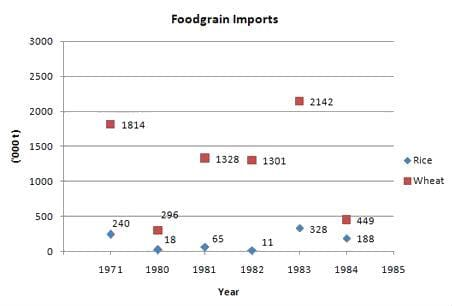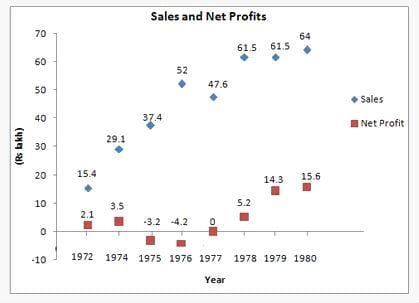GMAT  >  Scatter Plot Graph and Solved Examples

# Scatter Plot Graph and Solved Examples - Notes | Study Integrated Reasoning for GMAT - GMAT

 1 Crore+ students have signed up on EduRev. Have you?

The points which represent the relationship between the two sets of information.

Let us take an example of scattered plots:

Given that collected data about exams in each of her classes from the previous year.
Plot the data in a scatter plot.

 Class Math English History Computer Biology Arts Period 2 4 1 5 3 6 Average Score 95 60 75 85 92 83

Correlation

When the 2 sets of given data are strongly linked to each other, we can say they have a High Correlation.

Negative Correlation is when one value increases as the other decreases, and vice versa.

Positive Correlation is when both the values increase or decrease together.

Solved Examples

DIRECTIONS for question 1 to 3: Refer to the following graph and answer the questions given below:The total values of food grains (rice and wheat) imported during these years are given below:

1971 : Rs. 123 crore

1980 : Rs. 80 crore

1981 : Rs. 314 crore

1982 : Rs. 295 crore

1983 : Rs. 587 crore

1984 : Rs. 158 crore

Q 1.  Wheat formed what percent of the volume of total imports of food grains from 1980-84?

Option:
1. 75 %
2. 66 %
3. 90 %
4. 95 %

Solution: Option 3

Adding up the approximate quantity of wheat and dividing it by the total quantity of food grains

(i.e. wheat + rice) will give us

≈ 5516 / 6126 » 90 %.

Q 2.  If the import price of wheat was Rs. 2,400 per tonne in 1983, then what was the import price of rice per tonne during that year?

Option:
1. 3,200
2. 2,225
3. 2,850
4. 1,800

Solution: Option 2

In the year 1983, 2,142,000 tonnes of wheat @ Rs 2,400/tonne will mean an expenditure of  Rs 514 crore.

So the remaining is 587 – 514 = 73 crore, which was spent on importing 328,000 tonnes of rice. So the price of rice = 730,000 / 328 ≈ Rs 2,225 /tonne

Q 3.  In which year was the ratio of rice to wheat imports the highest?

Option:
1. 1971
2. 1980
3. 1983
4. 1984

Solution: Option 4

The ratio is maximum when the numerator is maximum and the denominator is minimum. In the year 1984 the imports of rice are highest and the imports of wheat is the least which gives the maximum ratio.

DIRECTIONS for question 4 to 6: Refer to the following graphQ 4. In which of the following years was the expenditure of the company the maximum?

1. 1980
2. 1979
3. 1978
4. 1976

Solution: Expenditure of the company is : Total Sales – Net Profit.

The difference of the total sales & net profit is highest for the year 1978.

Q 5. If a tax of 55 % is to be paid on gross profit before the net profit of that particular year is arrived at, what is the combined gross profit for the years ‘79 and ‘80? (' in Lakhs)

1. 32
2. 17
3. 46
4. 67

Solution: The combined Net Profit for both the years  79 and 80 was 29.9  ≈ 30 lakh.

This net profit is calculated  after having paid 55 % tax on the gross profit of that particular year.

So the remaining amount will be 45 % of the gross profit  and this equals  to  INR 30 lakh.

So 100 % of that amount, i.e. the combined gross profit must correspond to 30/45 x 100 = 66.66 » 67 lakh. Hence 4 option is the correct answer.

Q 6. If the expenses  on advertising the products  in 1980 was 2.5 % of the total sales turnover of the company, what was it as a percentage of the net profit in 1980?

1. 11.8%
2. 5.7%
3. 13.5%
4. 10.2%

Solution: The advertisement expenditure was 2.5 % of the total sales  turnover

= 2.5/100 x  64 = 1.6.

So the percentage of net Profit = 1.6/15.6 x 100

≈ 10(+) %. Hence 4th option is the correct answer.

The document Scatter Plot Graph and Solved Examples - Notes | Study Integrated Reasoning for GMAT - GMAT is a part of the GMAT Course Integrated Reasoning for GMAT.
All you need of GMAT at this link: GMAT

## Integrated Reasoning for GMAT

24 videos|33 docs|24 tests
 Use Code STAYHOME200 and get INR 200 additional OFF

## Integrated Reasoning for GMAT

24 videos|33 docs|24 tests

Track your progress, build streaks, highlight & save important lessons and more!

,

,

,

,

,

,

,

,

,

,

,

,

,

,

,

,

,

,

,

,

,

;